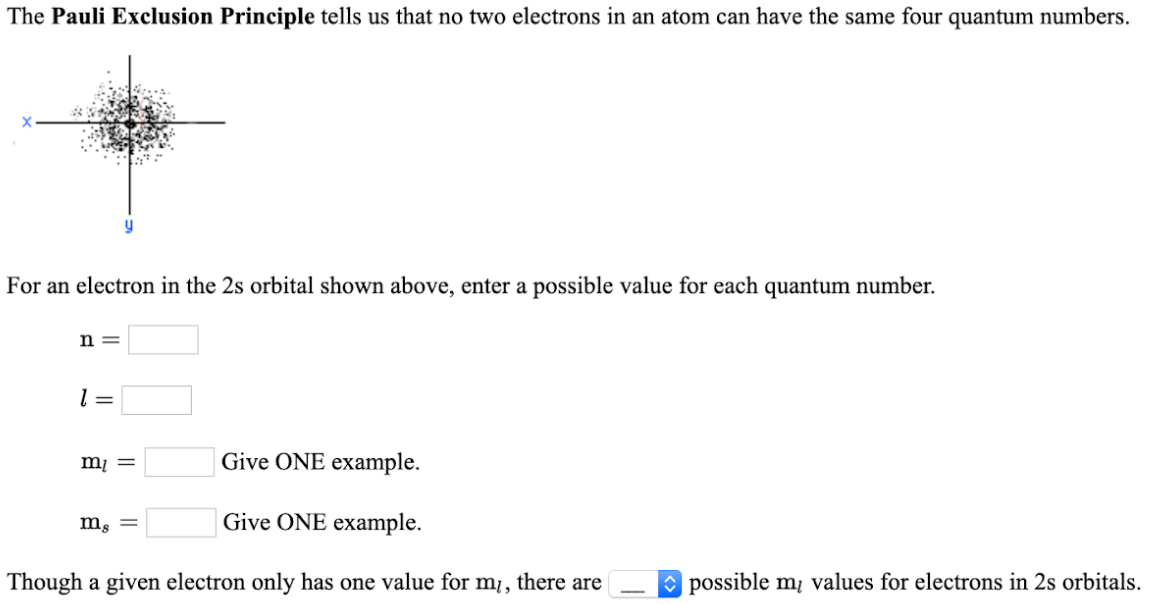# The Pauli Exclusion Principle tells us that no two electrons in an atom can have the same four quantum numbers. For an electron in the 2s orbital shown above, enter a possible value for each quantum number. n = ____ l = ____ ml = ____ Give ONE example. ms = ____ Give ONE example. Though a given electron only has one value for ml, there are ____ possible ml values for electrons in 2s orbitals.